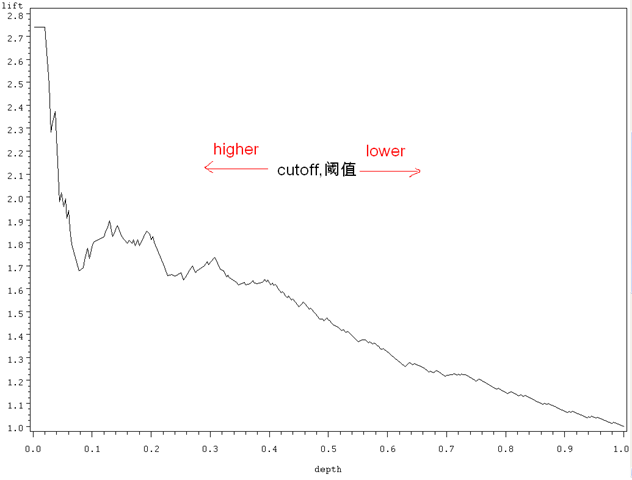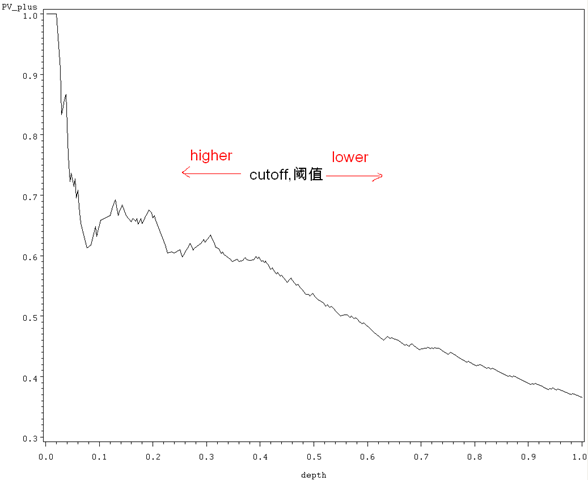# 一些准备

1 0
1 d, True Positive c, False Negative c+d, Actual Positive
0 b, False Positive a, True Negative a+b, Actual Negative
b+d, Predicted Positive a+c, Predicted Negative

1. Sensitivity（覆盖率，True Positive Rate）=正确预测到的正例数/实际正例总数 Recall (True Positive Rate，or Sensitivity) =true positive/total actual positive=d/c+d
2. PV+ (命中率，Precision, Positive Predicted Value) =正确预测到的正例数/预测正例总数 Precision (Positive Predicted Value, PV+) =true positive/ total predicted positive=d/b+d
3. Specificity (负例的覆盖率，True Negative Rate) =正确预测到的负例个数/实际负例总数 Specificity (True Negative Rate) =true negative/total actual negative=a/a+b

Ptp=proportion of true positives=d/a+b+c+d=(c+d/a+b+c+d)*(d/c+d) =pi1* Sensitivity，正确预测到的正例个数占总观测值的比例

Pfp=proportion of false positives=b/a+b+c+d= (a+b/a+b+c+d)*(b/a+b) = (1-c+d/a+b+c+d)*(1-a/a+b) = (1-pi1)*(1- Specificity) ，把负例错误地预测成正例的个数占总数的比例

Depth=proportion allocated to class 1=b+d/a+b+c+d=Ptp+Pfp，预测成正例的比例

PV_plus=Precision (Positive Predicted Value, PV+) = d/b+d=Ptp/depth，正确预测到的正例数占预测正例总数的比例

Lift= (d/b+d)/(c+d/a+b+c+d)=PV_plus/pi1，提升值，解释见下节。

``````%let pi1=0.365;

data valid_lift;

set valid_roc;

cutoff=PROB;

Ptp=&pi1*SENSIT;

Pfp=(1-&pi1)*1MSPEC;

depth=Ptp+Pfp;

PV_plus=Ptp/depth;

lift=PV_plus/&pi1;

keep cutoff SENSIT 1MSPEC depth PV_plus lift;

run;
``````

Lift chart是不同阈值下Lift和Depth的轨迹

Gains chart是不同阈值下PV+和Depth的轨迹

# Lift

Lift = (d/b+d)/(c+d/a+b+c+d)=PV_plus/pi1)，这个指标需要多说两句。它衡量的是，与不利用模型相比，模型的预测能力“变好”了多少。不利用模型，我们只能利用“正例的比例是c+d/a+b+c+d”这个样本信息来估计正例的比例（baseline model），而利用模型之后，我们不需要从整个样本中来挑选正例，只需要从我们预测为正例的那个样本的子集（b+d）中挑选正例，这时预测的准确率为d/b+d。

``````symbol i=join v=none c=black;

proc gplot data=valid_lift;

plot lift*depth;

run; quit;
``````# Gains

Gains (增益) 与 Lift （提升）相当类似：Lift chart是不同阈值下Lift和Depth的轨迹，Gains chart是不同阈值下PV+和Depth的轨迹，而PV+=lift*pi1= d/b+d（见上），所以它们显而易见的区别就在于纵轴刻度的不同：

``````symbol i=join v=none c=black;

proc gplot data=valid_lift;

plot pv_plus*depth;

run; quit;
``````# 总结和下期预告：K-S

Gains chart是不同阈值下PV+和Depth的轨迹（Lift与之类似），而ROC是sensitivity和1-Specificity的对应，前面还提到，Sensitivity（覆盖率，True Positive Rate）在欺诈监控方面更有用（所以ROC更适合出现在这个场合），而PV+在数据库营销里面更有用（这里多用Gains/Lift）。